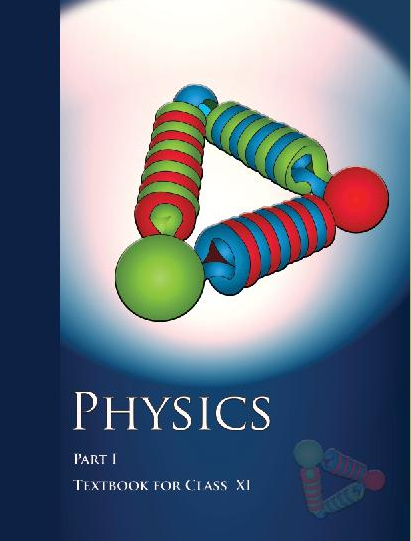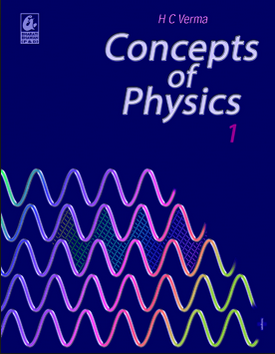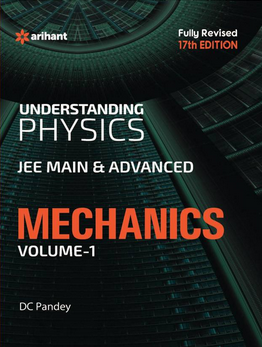# Work Energy and Power   Share

## What is Work Energy and Power

You must have heard these terms work, energy, and power very frequently in your day to day life, like a barber cutting hair, a laborer lifting bricks and transporting them and a student studying are all said to be working. But here in physics, work is defined using very specific words and it has a very precise meaning. Similarly, a gymnast who can do a lot number of pull-ups we say that he/she has very good stamina or in other words, he/she has a good amount of energy. This means energy is something which tells about the capacity to do the work. In physics as well energy is related to work similarly but as already mentioned the definition of the word Work is very precise itself in Physics hence the definition of energy as well.  We often come across the word Power as well, for example, the bodybuilder hit the punching bag with huge power, so in a very crude way, we could say that the rate of doing work is power. We could loosely correlate the physical definitions and physiological pictures. In this chapter, we would go in depth of the definitions and relations between these three physical quantities.## Work Energy and Power Topics

Work is done by a force (Both constant force and a variable force), conservation of mechanical energy, potential energy, kinetic energy, work-energy theorem, Potential energy of a spring, conservative and non-conservative forces, power

## Overview of Work Energy and Power

This chapter is very important because it will test your knowledge of the previous two chapters namely, Kinematics, Laws of Motion and it will be also useful and crucial for you for solving questions from the next chapters namely System of Particles, Rotational Motion and to some extent gravitation.

Prepare Online for JEE Main/NEET

Crack JEE 2021 with JEE/NEET Online Preparation Program

Here we will try to understand the definition of Work, Energy, and Power.

Work: It is defined as the product of the force applied to the object and the distance it travels in the direction which is parallel to the direction of the force. It is represented by the letter W. It is a scalar quantity. S.I. unit of work is N-m or J (joules).Energy: It is the capacity to do work, it has the same unit as work and also it is a scalar quantity. But energy exists in many forms like Kinetic energy, Potential energy, Thermal energy, Heat energy, etc, but here we will only talk about kinetic energy and potential energy. It is denoted by letter E.

Power: It is the rate of doing work or the rate of transferring energy, It is denoted by the letter P and its S.I. units are J/s or W (watts).You will also come across a very important theorem of this chapter, known as a work-energy theorem. This theorem states that the work done on the particle due to all types of forces equals to change in kinetic energy of the particles. Let’s understand this theorem with the help of an example.

Example - A bob-pendulum was hanging through a height ‘h’ from its equilibrium position as shown in the figure. How much velocity will it attain at its equilibrium position if it is dropped from the height ‘h’?Solution - We will take the help of Work energy theorem for solving this problem. Assuming the mass of the bob is ‘m’. The force which is acting on the bob is a gravitational force due to its weight.Its initial velocity is zero,## How to prepare Work Energy and Power

It is one of the basic and important chapters in mechanics because with the help of the concept used in this chapter you will be able to solve questions from other mechanics chapters as well. So for preparing this chapter, you need to remember the concept from laws of motion and you should be able to understand what kind of forces are acting on the particle. You should also understand how scalar product of the two vectors is operated and what is the significance of scalar product. Because work, energy, and power all are a scalar quantity.

## Notes for Work Energy and Power

• This chapter is more of a concept based chapter there is very little to memorize, hence practice is the key here for getting a good hold on this chapter.

• Some questions of this chapter will involve the use of calculus and Scalar product of vectors, hence keep your basics strong.

• Give a significant number of mock tests and make note of your progress.

• It would be easy for you to solve questions if you can imagine what all forces are acting on the object and how.

• Make a list of questions which you were not able to solve in the first attempt or you took a long time solving them, after a week or two solve them again.

## Work Energy and Power- Books

For this chapter, we would recommend you to first go through NCERT book and your Lab manual and solve questions from those chapters. Then you should solve questions from NCERT Exemplar book for a good hold on this chapter. If you want to test yourself for competitive exams, then you should read Understanding Physics by D.C. Pandey.

## Physics Chapter wise Notes For Engineering and Medical Exams

 Chapters No. Chapters Name Chapter 1 Physics and Measurement Chapter 2 Kinematics Chapter 3 Laws of motion Chapter 5 Rotational Motion Chapter 6 Gravitation Chapter 7 Properties of Solids and Liquids Chapter 8 Kinetic theory of Gases Chapter 9 Thermodynamics Chapter 10 Oscillations and Waves Chapter 11 Electrostatics Chapter 12 Current Electricity Chapter 13 Magnetic Effects of Current and Magnetism Chapter 14 Electromagnetic Induction and Alternating currents Chapter 15 Electromagnetic Waves Chapter 16 Optics Chapter 17 Dual Nature of Matter and Radiation Chapter  18 Atoms And Nuclei Chapter 19 Electronic devices Chapter 20 Communication Systems Chapter 21 Experimental skills

## How to prepare for Work Energy and Power for JEE Main

Overview of the chapter

To study this chapter efficiently first you have to understand all the definitions and terminologies, given the chapters and try to interpret them in a very logical way. You must be good at basic laws like the law of energy conservation and theorems like a work-energy theorem Because you might get conceptual questions based on these laws And theorems. You will get various real-life application based problems on the concept like a work-energy theorem, law of energy conservation, Collision, Power.
For example, let's have a look question which is based on real-life activities and was asked in jee main 2016 from this chapter.

Q-  A person who is trying to lose weight by burning his fats lifts a mass of 10 kg 1000 times up to a height of 1 m.  And also assume that the potential energy lost each time he lowers the mass is dissipated.  By considering the work done only when the weight is lifted up then tell How much fat will he use up? ( If Fat supplies 3.8× J of energy on burning 1 kg fat which is converted to mechanical energy with a 20% efficiency rate. )

To solve these questions you first find work done by the person in lifting the weight
Which is equal to W=mgh
Which is equal to  E

Then find Total fat burn by dividing W by E
Which is equal to

## How to Prepare

First, you should have good command over the concepts of Work Power and Energy and you should also know how to apply them well at the time of the entrance exam or while solving questions. Entrance 360 will help you to understand each concept from this chapter since it provides you theory, questions with solutions and video lectures on each important concepts. For each concept practice enough problems so that you are through with the concept. Take mock test and practice previous year questions. Through practice, you will come to know how to approach a question. There are direct formulas that can be applied to certain questions.

To Solve Work Power Energy Problems

• .Master the concept of Kinematics, Laws of motion, Centre Of Mass, Impulse, and Momentum. This will help you to understand applying force in the problems.
• After reading problems you must define a system and then identify all the forces in the system and energy going in and out.
• You should try to balance the kinetic energy, potential energy and the work done for the given problem. Pay special attention to questions on springs and try similar questions from HCV and  Entrance360 site.
• You must know all the formulas from this chapter, and use these formulas to find unknown variables.

## Tips

• Make a plan to prepare for the chapter and Stick to a Timetable, Entrance 360 knockout series will help you make customized time table according to available time for preparation.
• Go through all NCERT basics, the NCERT textbooks are very important and most advisable for understanding theory and it mostly covers the full syllabus.
• Study the concept first and then start solving the question. Don't go through question directly without knowing a concept.
• Solve previous year question paper.
• Take a practice test.
• Use smart methods to solve questions.

Books

For Work Power and Energy chapter concepts in NCERT is enough and practice questions which are asked in previous questions and you can follow other standard books available for JEE preparation like Concepts of Physics (H. C. Verma) and Understanding Physics by D. C. Pandey (Arihant Publications). You don't have to study the whole book to understand the concept from this chapter because Entrance 360 will provide you exact page number and line number of these books where you will get these concepts to read.

### Topics from Work Energy and Power

• Work done by force ( JEE Main, KCET, TS EAMCET, AP EAMCET, AP EAMCET Pharmacy, TS EAMCET Pharmacy, COMEDK UGET ) (60 concepts)
• Kinetic and Potential Energy ( JEE Main, KCET, TS EAMCET, AP EAMCET, AP EAMCET Pharmacy, TS EAMCET Pharmacy, COMEDK UGET ) (24 concepts)
• Potential energy of a spring ( JEE Main, KCET, TS EAMCET, AP EAMCET, AP EAMCET Pharmacy, TS EAMCET Pharmacy, COMEDK UGET ) (4 concepts)
• Work Energy Theorem ( JEE Main, KCET, TS EAMCET, AP EAMCET, AP EAMCET Pharmacy, TS EAMCET Pharmacy, COMEDK UGET ) (8 concepts)
• Conservation of energy ( JEE Main, KCET, TS EAMCET, AP EAMCET, AP EAMCET Pharmacy, TS EAMCET Pharmacy, COMEDK UGET ) (12 concepts)
• Power ( JEE Main, KCET, TS EAMCET, AP EAMCET, AP EAMCET Pharmacy, TS EAMCET Pharmacy, COMEDK UGET ) (16 concepts)
• Elastic and Inelastic Collisions ( JEE Main, KCET, TS EAMCET, AP EAMCET, AP EAMCET Pharmacy, TS EAMCET Pharmacy, COMEDK UGET ) (44 concepts)
• Work ( JEE Main, KCET, TS EAMCET, AP EAMCET, AP EAMCET Pharmacy, TS EAMCET Pharmacy, COMEDK UGET ) (20 concepts)
• Energy and Power ( JEE Main, KCET, TS EAMCET, AP EAMCET, AP EAMCET Pharmacy, TS EAMCET Pharmacy, COMEDK UGET ) (32 concepts)
• Collision ( JEE Main, KCET, TS EAMCET, AP EAMCET, AP EAMCET Pharmacy, TS EAMCET Pharmacy, COMEDK UGET ) (28 concepts)

### Important Books for Work Energy and Power

•••Exams
Articles
Questions